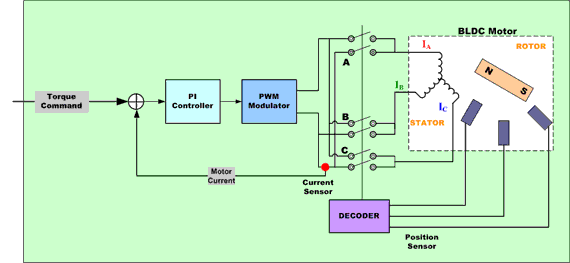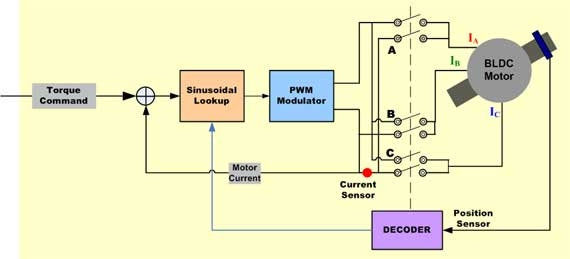Brushless motors are not self-commutating, and hence are more complicated to control.

BLDC motor control requires knowledge of the rotor position and mechanism to commutate the motor. For closed-loop speed control there are two additional requirements, measurement of the motor speed and/or motor current and PWM signal to control the motor speed and power.

BLDC motors can use edge-aligned or center-aligned PWM signals depending on the application requirements. Most applications, that only require variable speed operation, will use six independent edge-aligned PWM signals. This provides the highest resolution. If the application requires servo-positioning, dynamic braking, or dynamic reversal, it is recommended that complementary center-aligned PWM signals be used.

To sense the rotor position BLDC motors use Hall Effect sensors to provide absolute position sensing. This results in more wires and higher cost. Sensorless BLDC control eliminates the need for Hall effect sensors, using the back-EMF (electromotive force) of the motor instead to estimate the rotor position. Sensorless control is essential for low-cost variable speed applications such as fans and pumps. Refrigerator and air conditioning compressors also require sensorless control when using BLDC motors.

## Dead Time Insertion and Compensation

Many different control algorithms have been used to provide control of BLDC motors. Typically, the motor voltage is controlled using a power transistor operating as a linear voltage regulator. This is not practical when driving higher-power motors. High-power motors must use PWM control and require a microcontroller to provide starting and control functions.

The control algorithm must provide three things:

• PWM voltage to control the motor speed
• Mechanism to commutate the motor
• Method to estimate the rotor position using the back-EMF or Hall Sensors

Pulse-width modulation is used to apply a variable voltage to the motor windings. The effective voltage is proportional to the PWM duty cycle. When properly commutated, the torque-speed characteristics of the BLDC motor are identical to a dc motor. The variable voltage can be used to control the speed of the motor and the available torque.

The commutation of the power transistors energizes the appropriate windings in the stator to provide optimum torque generation depending on the rotor position. In a BLDC motor, the MCU must know the position of the rotor and commutate at the appropriate time.

### Trapezoidal Commutation of BLDC Motor

One of the simplest methods of control for dc brushless motors uses what is termed Trapezoidal commutation.Figure 1: Simplified Block Diagram of Trapezoidal Controller for BLDC Motor

In this scheme, current is controlled through motor terminals one pair at a time, with the third motor terminal always electrically disconnected from the source of power.

Three Hall devices embedded in the motor are usually used to provide digital signals which measure rotor position within 60 degree sectors and provide this information to the motor controller. Because at any time, the currents in two of the windings are equal in magnitude and the third is zero, this method can only produce current space vectors having one of six different directions. As the motor turns, the current to the motor terminals is electrically switched (commutated) every 60 degrees of rotation so that the current space vector is always within the nearest 30 degrees of the quadrature direction.

The current waveform for each winding is therefore a staircase from zero, to positive current, to zero, and then to negative current.

This produces a current space vector that approximates smooth rotation as it steps among six distinct directions as the rotor turns.

In motor applications such as air conditioners and refrigerators use of Hall-Effect sensors is not a viable option. Back-EMF sensors that sense the back EMF in the unconnected winding can be used to achieve the same results

The trapezoidal-current drive systems are popular because of the simplicity of their control circuits but suffer from a torque ripple problem during commutation.

## Sinusoidal Commutation for BDLC Motors

Trapezoidal commutation is inadequate to provide smooth and precise motor control of brushless dc motors, particularly at low speeds. Sinusoidal commutation solves this problem. This is because the torque produced in a three phase brushless motor (with a sine wave back-EMF) is defined by the following equation:

Shaft Torque = Kt [ IR Sin(o) + IS Sin(o+120) + IT Sin(o+240)]; where:

• o is the electrical angle of the shaft,
• Kt is the torque constant of the motor and
• IR , IS and IT are the phase currents.

If the phase currents are sinusoidal: IR = I0 Sino; IS = I0 Sin (+120o); IT = I0 Sin (+240o); this reduces to:

• Shaft Torque = 1.5I 0 * Kt (a constant independent of the shaft angle)

Sinusoidally commutated brushless motor controllers attempt to drive the three motor windings with three currents that vary smoothly and sinusoidally as the motor turns. The relative phases of these currents are chosen so that they should result in a smoothly rotating current space vector that is always in the quadrature direction with respect to the rotor and has constant magnitude. This eliminates the torque ripple and commutation spikes associated with trapezoidal commutation.

In order to generate smooth sinusoidal modulation of the motor currents as the motor turns, an accurate measurement of rotor position is required. The Hall devices provide only a coarse measure of rotor position and are inadequate for this purpose. For this reason, angle feedback from an encoder, or similar device, is required.Figure 3: Simplified Block Diagram of Sinusoidal Controller for BLDC Motor

Since the winding currents must combine to produce a smoothly rotating current space vector of constant magnitude, and because the stator windings are oriented 120 degrees apart from each other, currents in each winding must be sinusoidal and phase shifted by 120 degrees. Position information from the encoder is used to synthesize two sinusoids, one 120 degrees phase shifted from the other. These signals are then multiplied by the torque command so that the amplitudes of the sine waves are proportional to desired torque. The result is two sinusoidal current command signals appropriately phased to produce a rotating stator current space vector in the quadrature direction.

The sinusoidal current command signals are provided as inputs to a pair of P-I controllers that regulate current in the two appropriate motor windings. The current in the third motor winding is the negative sum of the currents in the controlled windings and therefore cannot be separately controlled. The output from each P-I controller is fed to a PWM modulator and then to the output bridge and two motor terminals. Voltage applied to the third motor terminal is derived as the negative sum of the signals applied to the first two windings, as appropriate for three sinusoidal voltages each separated by 120 degrees.

To the extent that the actual output current waveform accurately tracks the sinusoidal current command signals, the resulting current space vector is smoothly rotating, constant in magnitude and oriented in the quadrate direction as desired.

Sinusoidal commutation results in the smoothness of control that is generally unachievable with trapezoidal commutation. However, while it is very effective at low motor speeds, it tends to fall apart at high motor speeds. This is because as speed goes up the current loop controllers must track a sinusoidal signal of increasing frequency. At the same time, they must overcome the motor back-EMF that also increases in amplitude and frequency as speed goes up.

Because the P-I controllers have limited gain and frequency response, the time-variant perturbations to the current control loop cause phase lag and gain error in the motor currents. Higher speeds result in larger errors. This perturbs the direction of the current space vector relative to the rotor, causing it to shift away from the quadrature direction.

When this happens, less torque is produced by a given amount of current and therefore more current is required to maintain torque. Efficiency deteriorates.

This degradation continues as speed increases. At some point, motor current phase shift crosses through 90 degrees. When this happens torque is reduced to zero. With sinusoidal commutation, speeds above this point result in negative torque and are therefore not achievable.

 Control Methods MCU Requirements Suggested MCUs RL78 Family RX Family RH850 Family RZ/T1 Group BLDC Motor Control Trapezoidal Drive Control Input Capture, Interrupt, PWM Timer for Three-Phase motor control ● Sinusoidal Drive Control Input Capture, Interrupt, PWM Timer for Three-Phase motor control ● ● Simplified Vector Control Input Capture, Interrupt, PWM Timer with Deadtime for Three-Phase motor control ● ● ● Vector Control(FOC) High Performance MCU＋MAC、High-Speed A/D Converter, Input Capture, Interrupt, PWM Timer with Deadtime for Three-Phase motor control ● ● ● Sensorless Trapezoidal Drive Control Input Capture, High-Speed A/D Converter, Interrupt, PWM Timer for Three-Phase motor control ● Sensorless Vector Control High Performance MCU＋MAC、 High-Speed A/D Converter, Interrupt, PWM Timer with Deadtime for Three-Phase motor control ● ● ●

Title Type Date
Application Note